# 高级数据库系统 03：容错基础知识

## 墨尔本大学 COMP90050 课程笔记

Posted by YEY on July 8, 2020

# Lecture 03 容错基础知识

## 1. 概率基础

• $P(A)=$ 事件 $A$ 在 特定时期内 发生的概率

• $P(A \cap B)=$ 在这段时期内事件 $A$ 和 $B$ 都发生的概率
如果 $A$ 和 $B$ 互为独立事件，那么

$P(A \cap B)=P(A)\times P(B)$

• $P(A \cup B)= P(A) + P(B) - P(A\cap B)$
如果 $A$ 和 $B$ 互为独立事件，那么

\begin{align} P(A \cup B) &= P(A)+P(B)-P(A)\times P(B) \\ &= P(A)+P(B)\qquad (如果\, P(A)\, 和\, P(B)\, 非常小)\end{align}

• 事件发生的平均时间 $MT(A)=1/P(A)$

• 如果事件 $A$ 和 $B$ 的平均时间分别为 $MT(A)$ 和 $MT(B)$，那么到第一次事件发生的平均时间为

$MT(A\cup B)=\dfrac{1}{P(A\cup B)}=\dfrac{1}{P(A)+P(B)-P(A\cap B)}$

假设 $P(A)$ 和 $P(B)$ 都非常小，那么我们可以认为 $P(A\cap B)\approx 0$。因此，在容错问题中，我们可以认为两个系统同时发生故障的概率非常低，此时

$MT(A\cup B)=\dfrac{1}{P(A)+P(B)}$

## 2. 可用性和失效率

### 2.1 模块可用性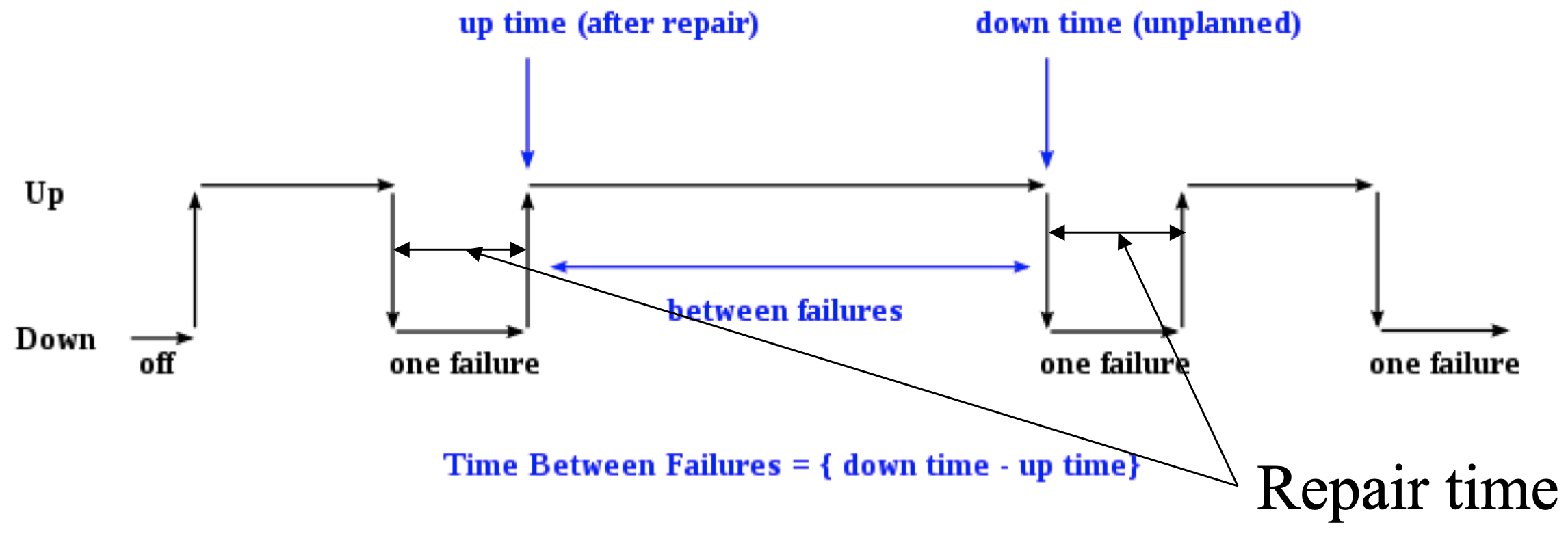$\dfrac{\text{Mean time to failure}}{\text{Mean time to failure} + \text{mean time to repair}}$

### 2.2 事件发生平均时间

$\dfrac{1}{np}=\dfrac{1}{p}\times \dfrac{1}{n}=\dfrac{m}{n}$

### 2.3 基于可用性的系统分类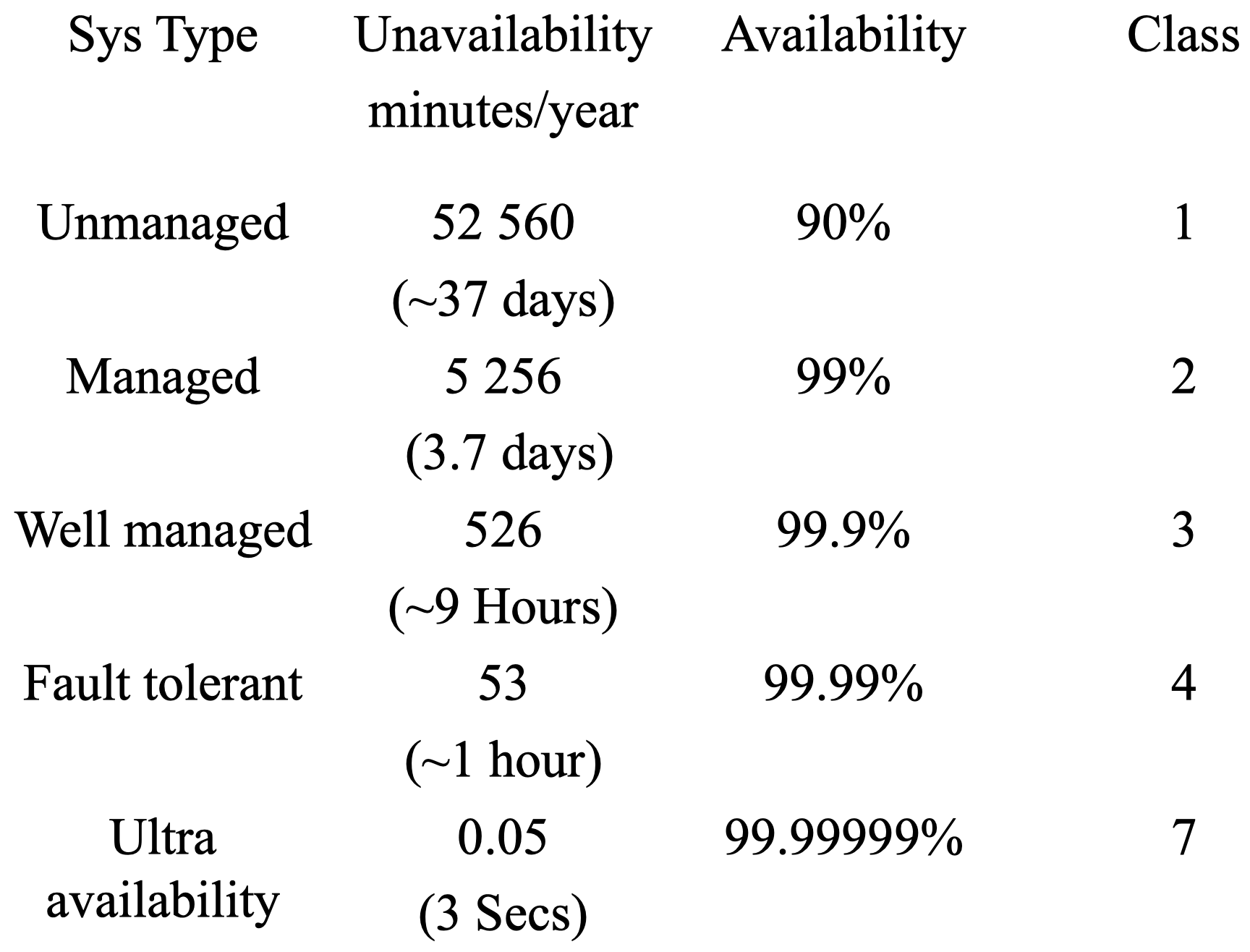### 2.4 影响系统可用性的因素

• 环境方面：诸如冷却、电源、天气、数据通信线路、火灾、地震、海啸、战争、人为破坏等
• 操作方面：系统管理、系统配置和系统操作程序
• 维护：定期维护的程序、定期更换硬件
• 硬件：设备、冷却
• 软件：程序
• 流程：罢工、宕机管理决策
• 内战

### 2.5 失效率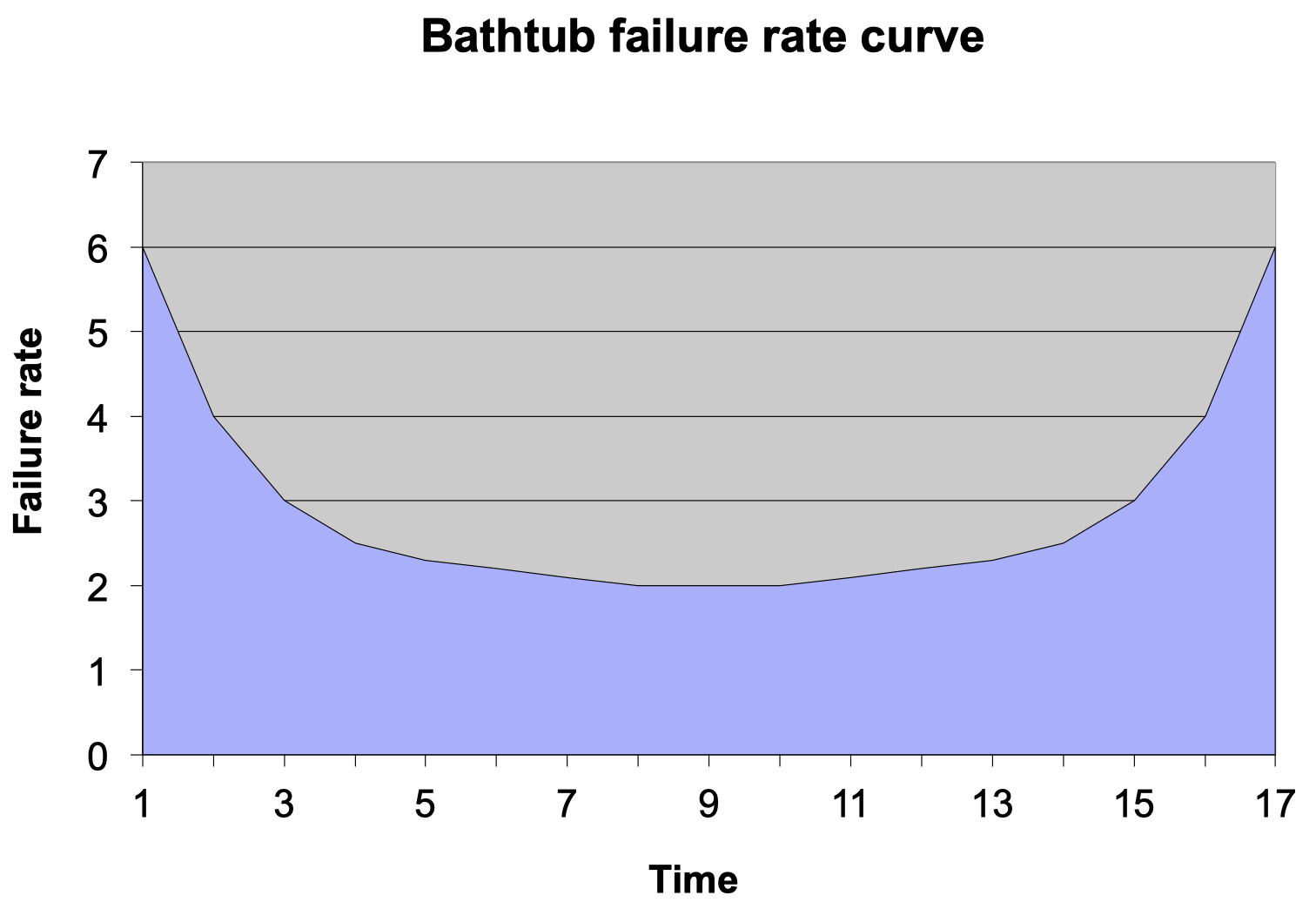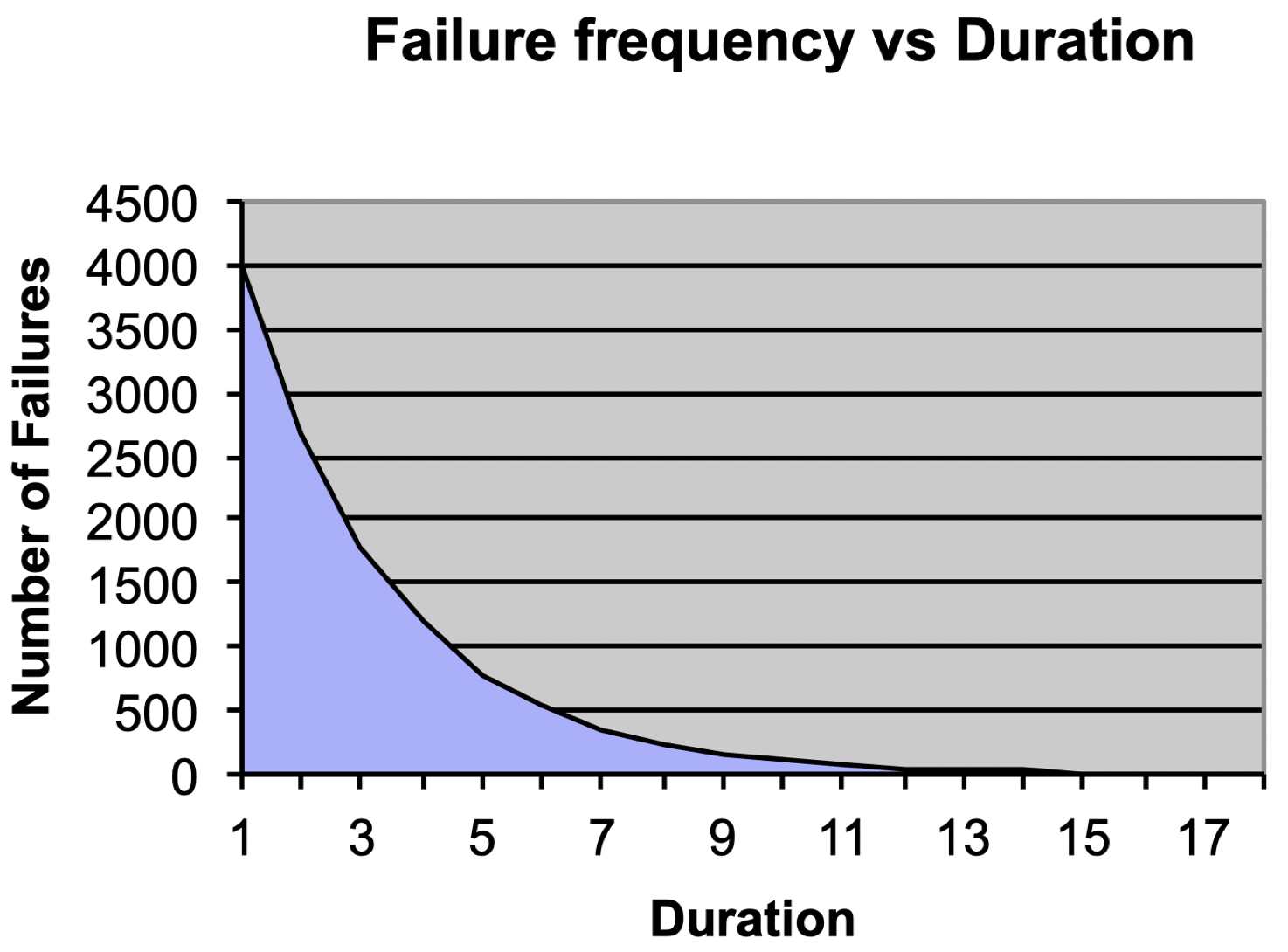### 2.6 磁盘失效率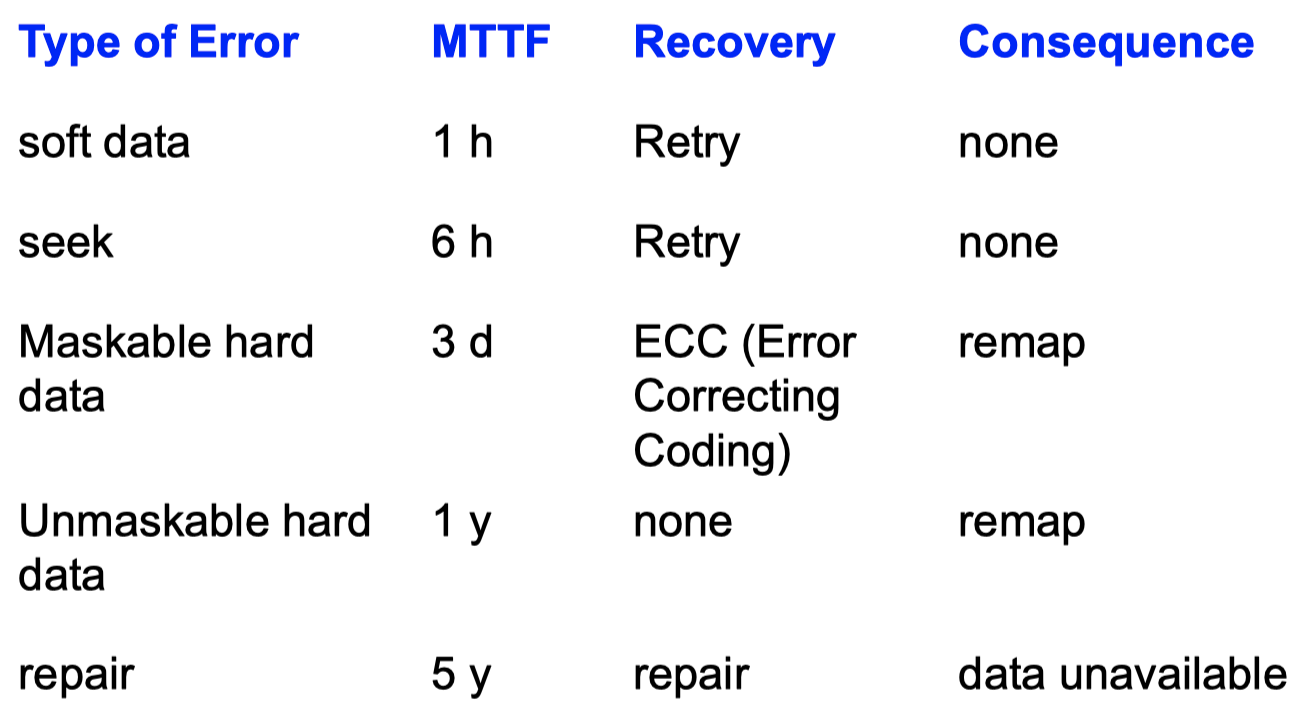## 3. 投票 (Voting)

### 3.1 多数投票

• Failvote：在 所有模块 上实行多数表决。例如：对于 3-plex 系统，多数意味着 $2/3$；对于 5-plex 系统，多数意味着 $3/5$。

Failvote 使用两个或多个模块并比较其输出。如果没有多数输出达成一致，则停止。尽管包含重复 (一对) 模块的失败次数是单模块失败次数的两倍，但其提供了清晰的失败语义。

• 通过三工化，系统的 MTTF 降至单个模块的为 $5/6 = 1/3 + 1/2 = 0.83$

令 $M$ 为单个模块的 MTTF。三工系统中的任意一个模块的 MTTF 均为 $M/3$，然后，两个工作模块中的任意一个模块的 MTTF 为 $M/2$。整个系统总的 MMTF 为：

$M \times(1/3 + 1/2)= 0.83M$
• Failfast (voting)：此方案类似于 Failvote，区别在于 Failfast 系统会先检测哪些模块可用，然后在这些 可用模块 上实行多数表决。例如：对于 5-plex 系统，如果其中 2 个模块失效，这种情况下，系统的多数表决只需要剩下 3 个可用模块中的 2 个达成一致即可。

• 对于一个 10-plex Failfast 系统，直到其中 9 个模块都发生故障之前，它都将继续运行；而对于同样模块数的 Failvote 系统，当 5 个模块出现故障时，系统将停止运行。

• Failfast 系统的可用性要优于 Failvote 系统 (因为在没有多数同意的情况下，后者将停止运行)。

• 对于一个双工 (Duplex) 系统：$MTTF = 10/2 = 5$ 年。

• 对于一个三工 (Triplex) 系统：$MTTF = 10/3$ (第一次故障) $+ 10/2$ (第二次故障) $= 8.3$ 年。

• 如果三工系统可以屏蔽所有瞬态/软故障，并且硬故障的 MTTF 为 $100$ 年，则系统的 MTTF 为:

$100 \times（1/3 + 1/2）= 83.7 \text{ 年}$

### 3.2 Failvote 策略

Failvote 需要 全部设备中的多数 同意才能接受某项操作 (例如：读/写)

• 在三工系统中，我们有 3 台设备，我们至少需要其中 2 台设备同意才能继续进行操作。如果设备无法正常工作，并且我们没有多数表决，那么系统将停止。

• 方案 A：所有设备的都工作，所有设备都同意，那么接受操作（$3/3$ 同意）

• 方案 B：其中两台设备正常工作，并且这两台设备都同意，那么接受操作（$2/3$ 同意）

• 方案 C：其中两台设备正常工作，但这两台设备都不同意，那么不接受该操作，因为同意占比没有达到 $2/3$。

• 如果我们有 10 台设备，那么需要其中 6 台能够工作并就该决定达成共识，才能够继续运行。而当第 5 台设备失效时，系统将停止，因为剩余的能够进行同意表决的工作设备不足 6 台。

### 3.3 Failfast 策略

• 0 台设备有故障，我们有 10 台设备在工作，至少需要 6 台同意才能接受操作
• 1 台设备有故障，我们有 9 台设备在工作，至少需要 5 台同意才能接受操作
• 2 台设备有故障，我们有 8 台设备在工作，至少需要 5 台同意才能接受操作
• 3 台设备有故障，我们有 7 台设备在工作，至少需要 4 台同意才能接受操作
• 4 台设备有故障，我们有 6 台设备在工作，至少需要 4 台同意才能接受操作
• 5 台设备有故障，我们有 5 台设备在工作，至少需要 3 台同意才能接受操作
• 6 台设备有故障，我们有 4 台设备在工作，至少需要 3 台同意才能接受操作
• 7 台设备有故障，我们有 3 台设备在工作，至少需要 2 台同意才能接受操作
• 8 台设备有故障，我们有 2 台设备在工作，那么需要这 2 台都同意才能接受操作
• 9 台设备有故障，我们有 1 台设备在工作，那么系统将停止，因为没有可用的多数表决

### 3.4 相关概率

N-plex 修复：在这种配置下，设备故障一旦被检测到，其修复的平均时间为 MTTR (平均修复时间)。有时 MTTR 只是需要更换设备的时间。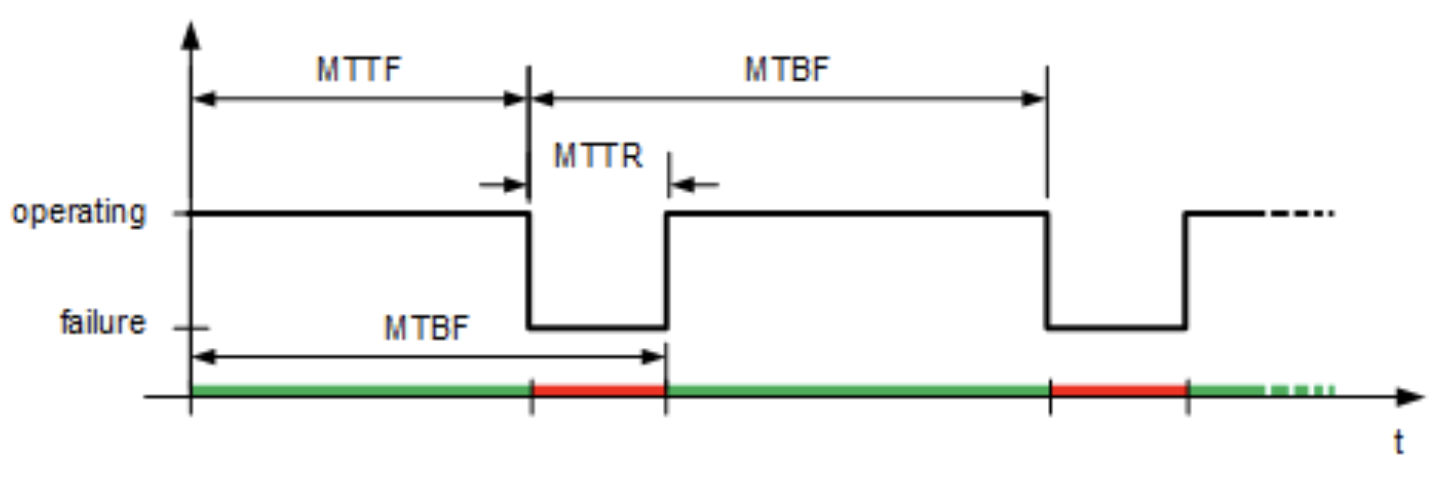• $MTTR =$ 几小时 (假设我们有备用磁盘) 到 1 天
• $MTTF =$ 750000 小时 (大约 86 年)

$P_1 = \dfrac{MTTR}{MTTF + MTTR}\cong \dfrac{MTTR}{MTTF} \quad (\text{如果}\; MTTF \gg MTTR)$

$P_{n-1}=\left(\dfrac{MTTR}{MTTF}\right)^{n-1}$

$P_f = \dfrac{1}{MTTF}$

$P_f \cdot P_{n-1}= \left(\dfrac{1}{MTTF}\right) \cdot \left(\dfrac{MTTR}{MTTF}\right)^{n-1}$

$P_{n-plex} = \left(\dfrac{n}{MTTF}\right) \cdot \left(\dfrac{MTTR}{MTTF}\right)^{n-1}$

$MTTF_n = \left(\dfrac{MTTF}{n}\right) \cdot \left(\dfrac{MTTF}{MTTR}\right)^{(n-1)}$

## 4. Old-New Master

• 将要执行的所有更新 (事务) 记录在单独的文件 (稳定存储) 中
• 在晚上 (通常) 使用旧 (前一天) 的母版和批量更新 (事务) 生成一个单独的新 (第二天) 母版。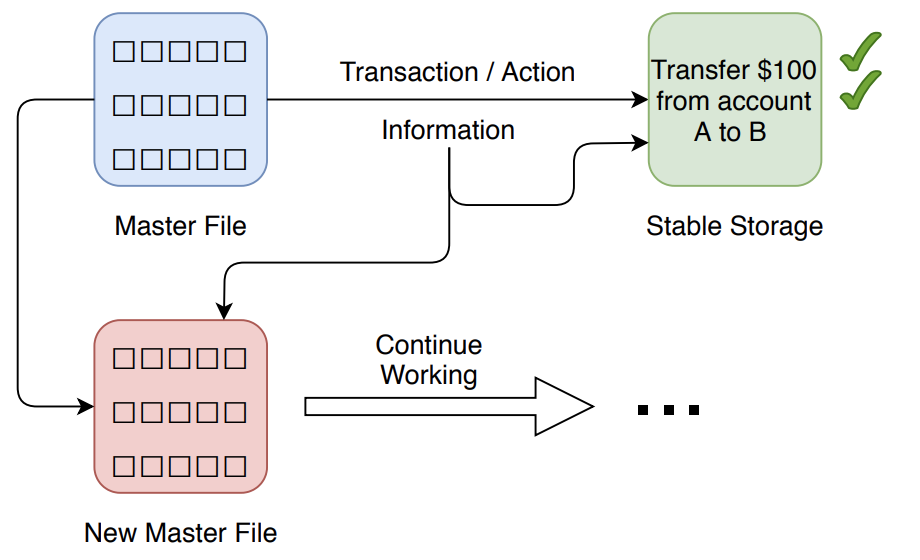## 5. 软件可靠性

• 硬件可靠性要求容忍 组件 故障。
• 软件可靠性要求容忍 设计和编码 错误。
• 硬件和软件之间的区别越来越小，因为大多数硬件单元都具有大量的软件组件。这些系统通常称为 嵌入式系统 (embedded systems)

### 5.1 N 版本程序设计

N 版本程序设计 (N-version programming)：为程序设计 $n$ 个版本，仔细地测试每个版本，然后并行地运行所有 $n$ 个版本的程序，选择 $n$ 个结果中占大多数的作为最终结果，利用这种设计差异就能够屏蔽许多故障。

• 使用 n 个并行运行的程序，对每个答案进行多数表决
• 优点是设计和编码的多样性可以屏蔽许多故障

### 5.2 事务

• 每个程序都被编写为带有一致性检查的 ACID 状态事务。
• 在没有适当恢复 (修复) 的情况下重新启动应用程序会使系统非常不可靠。
• 甚至一个事务处理系统也无法容忍应用程序错误。
• Bohrbug：以物理学家 Niels Bohr 命名，这些是确定性的 bug，相对容易处理。
• Heisenbug：以物理学家 Werner Heisenberg 命名，这些 bug 是偶然出现的瞬时 (非确定性) 软件错误，通常与负载条件和定时 (竞争条件) 有关。

### 5.3 故障

• 稠密故障 (Dense Faults)：算法是 $n$ 容错，系统在一段时间内最多可以容忍 $n$ 个故障。如果故障数超过 $n$，则系统可能会中断服务。

• 拜占庭式故障 (Byzantine Faults)：这类故障不是模型的一部分，并且在设计时没有考虑到容忍此类故障。例如：设计模型时一般不考虑诸如地震之类的环境问题。

### 5.4 如何提高软件可靠性

• 检查点重启 (Checkpoint-restart)：主进程将自己的状态记录在一个双工存储模块上。在接管时，后备进程从双工存储中读取主进程状态，然后恢复应用程序。

• 检查点消息 (Checkpoint messages)：主进程将其状态更改作为消息发送给后备进程。 接管时，备份将从最近的检查点消息中获取其当前状态。

• 持久进程 (Persistent)：后备进程以空状态重新启动，并允许事务处理机制清除所有尚未提交的事务。这是大部分数据库系统所采用的方法。

• 写入多个存储模块
• 具有某种校验和，以确保以很高的概率正确读取数据
• 磁盘镜像就是一个例子
• 阴影化是另一种镜像技术，允许原子写操作

• 进程配对
• 基于事务的重启
• 检查点重启

## 6. 如何提高通信可靠性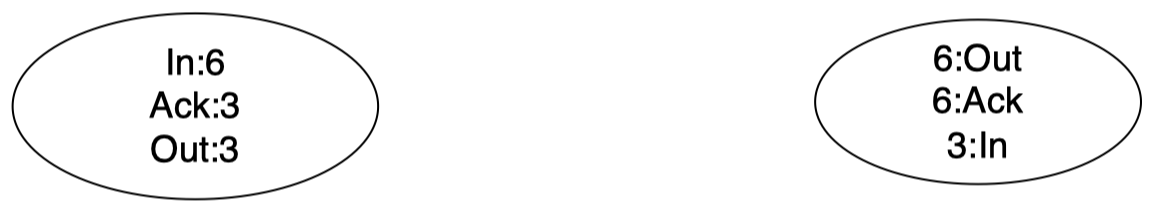• Out $=$ number of messages sent
• In $=$ number of messages received
• Ack $=$ number of acknowledgements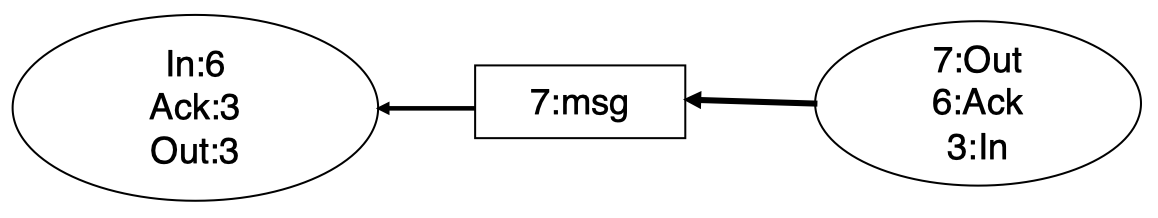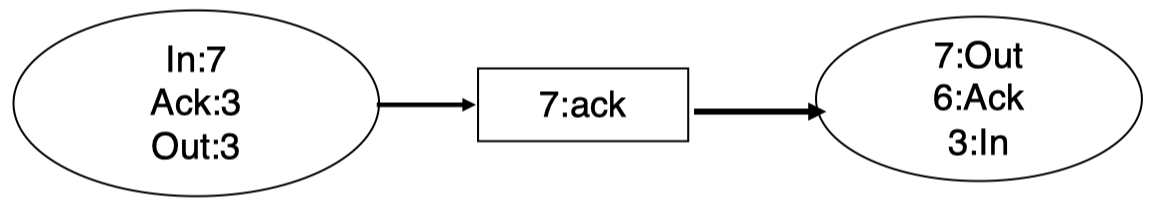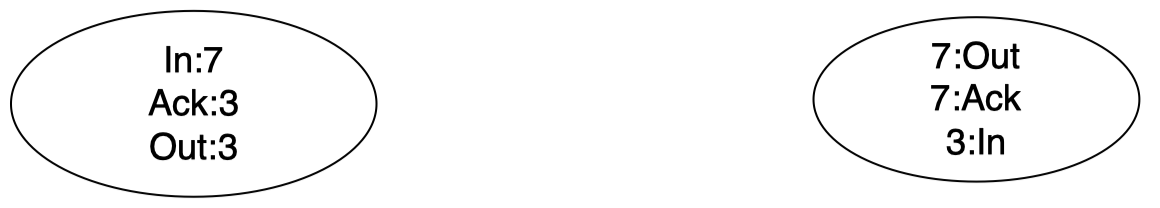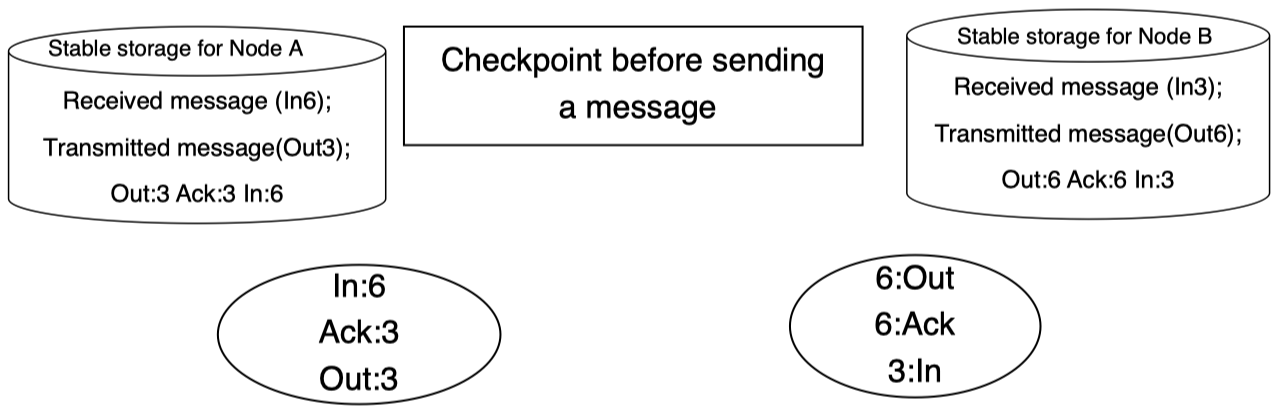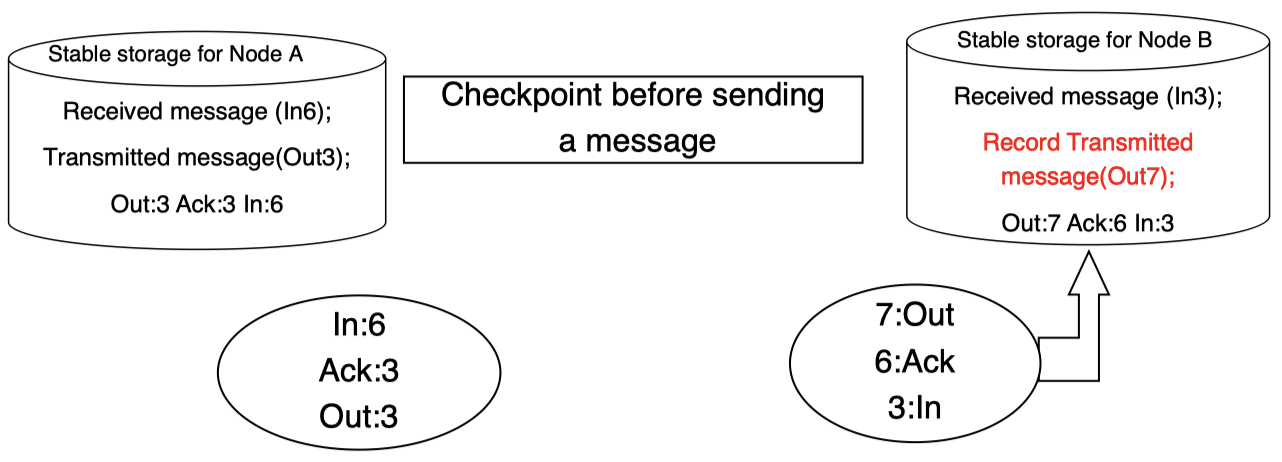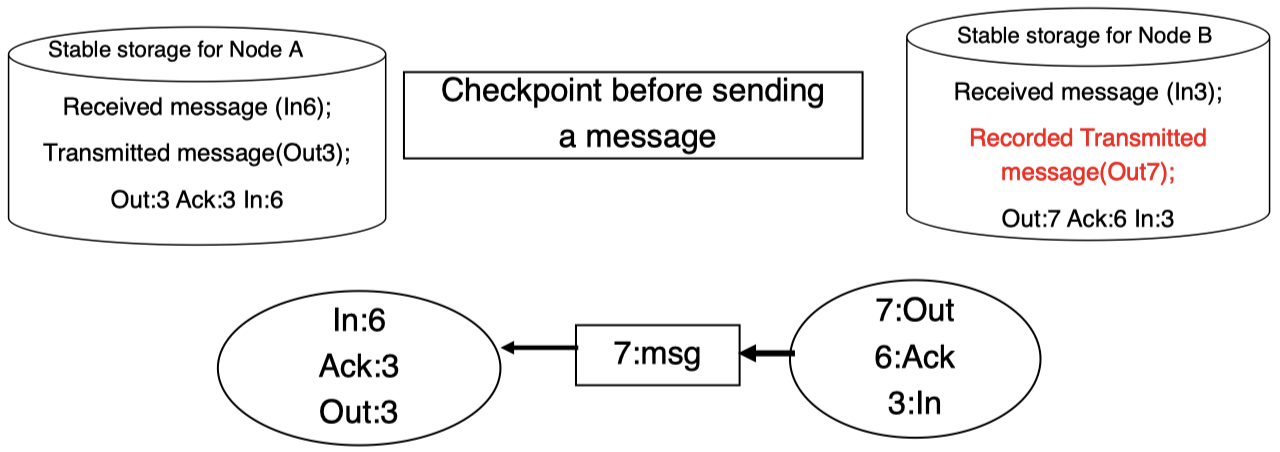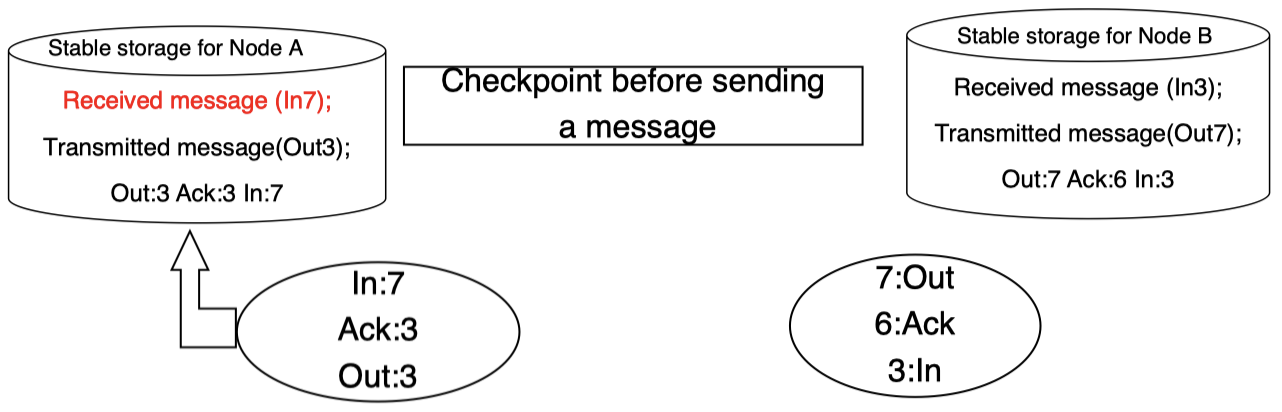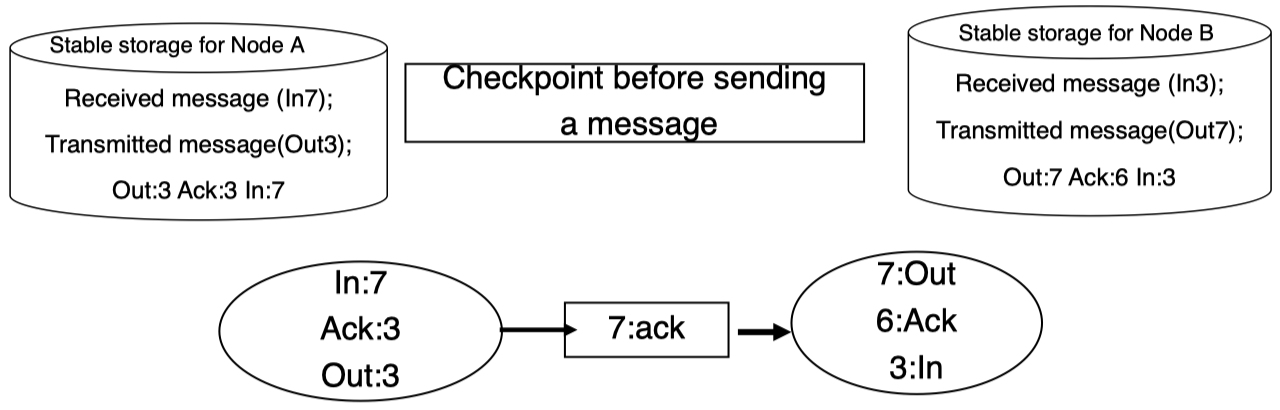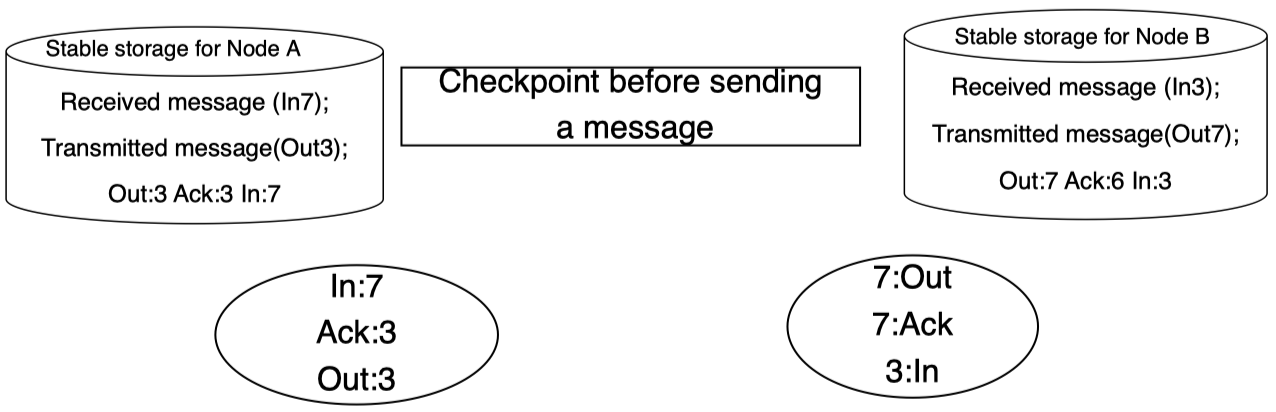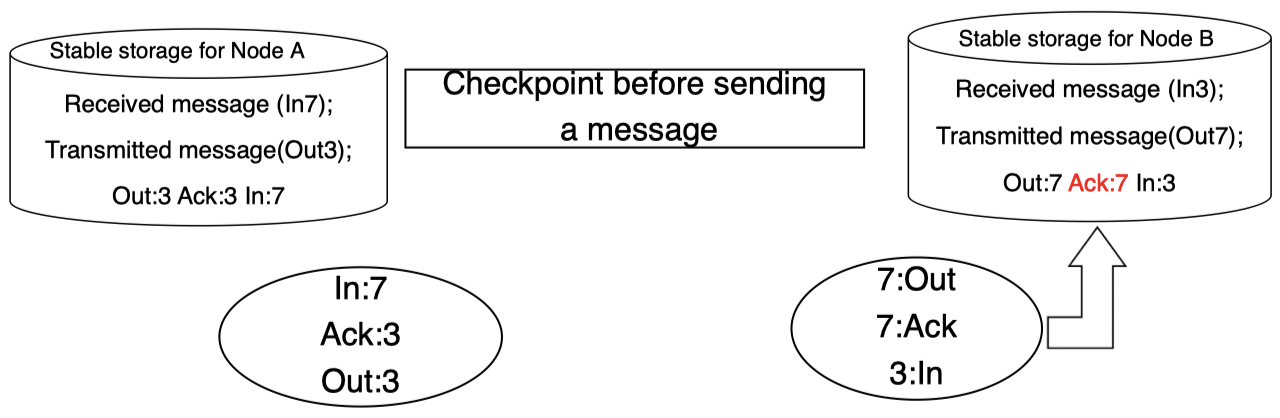## 7. 总结

• 提高 CPU、内存和存储单元的硬件可靠性。这可以通过采用大量冗余实现，例如：N-plex 系统。
• 通过采用进程对或者基于事务的恢复，可以提高软件可靠性。
• 通信系统的可靠性不仅需要硬件冗余，而且还需要保证消息的发送和接收，这可以通过使用稳定存储，以及采用重传和重复确认机制实现：直到消息被传递和确认为止。本作品采用知识共享署名-非商业性使用-相同方式共享 4.0 国际许可协议进行许可。 欢迎转载，并请注明来自：YEY 的博客 同时保持文章内容的完整和以上声明信息！